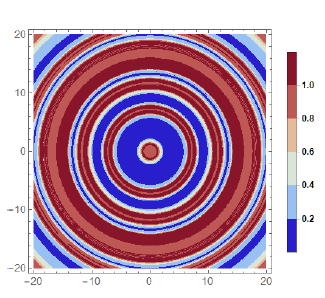# Master Theses in Mathematical Modelling and Industrial Mathematics at Sofia UniversityArguably, one of the most important parts of the education in a MSc program in Technomathematics is writing a Master thesis. We shall briefly present here four theses that were recently defended at the MSc program “Computational Mathematics and Mathematical Modelling” at the Faculty of Mathematics and Informatics, Sofia University.

Cloth Simulation, written by Dimitar Trendafilov

Cloths are everywhere, they comprise everything one wears and many items one touches on a daily basis. Therefore, one would suspect there are well established models we can use to simulate them, but actually this is not the
case. In fact cloth simulation is notoriously hard both computationally and mathematically. All available design and modeling tools have narrow usages and are specific to a problem area.

The thesis discusses two general approaches for the problem. First, models formulated in terms of systems of ODEs and based on a description of the fabric as a spring-mass system are considered. Then, computational models based on continuum mechanics and solved with the finite element method are analyzed.

As a result of the MSc thesis, a cloth simulation library in C++ was developed. which can be further extended and developed.

Computer Simulations of Demyelination Patterns in Multiple Sclerosis, written by Alexander Manov

The MSc thesis is devoted to mathematical modelling and computer simulations of the process of demyelination of a nerve cell, related to the progression of multiple sclerosis. A mathematical model, known from the literature, proposed by Lombardo et al. in 2017 is considered. It comprises of three non-linear reaction-diffusion-chemotaxis partial differential equations that describe the evolution of the three main agents in the process — the so-called macrophages, cytokines, and oligodendrocytes.

Using the assumption of radial symmetry, a 1D model of the process is derived. In order to study it numerically, two finite difference schemes are constructed and compared. Then, numerical experiments are carried out in order to study the process qualitatively. The pattern formations, obtained for appropriate values of the model parameters, are simulated. The effect of introducing a non-linear diffusion coefficient is studied. For several specific sets of parameter values, it is shown that new, irregular, patterns can be formed as a result of the non-linear coeficient.

Numerical simulations of the process of adsorption onto activated carbon in water treatment applications, written by Maria Zarcheva

Mathematical modelling and computer simulations are a powerful tool for the exploitation and management of water resources—an important current topic in environmental and engineering science. The Master’s thesis is concerned with
modelling water treatment using adsorption onto activated carbon filters—a widely used technology, whose proper management is essential for its effectiveness. A relatively simple mathematical model has been recently developed by a
Dutch water cycle research institute. It was presented at a European Study Group with Industry in the beginning of 2019. It comprises of 2N non-linear advection–adsorption partial differential equations, where N is the number of
contaminants in the water. The numerical solution of this model, however, was identified as a current problem for various values of the model parameters. The main goal of the Master’s thesis is the construction of a robust and
efficient numerical scheme for solving the model. To this end, two finite difference schemes are derived and compared. One of them meets the requirements of robustness and efficiency, as validated for a wide range of model parameters,
expected to be encountered in practice. This scheme is used in the thesis to carry out various numerical experiments that aim to simulate qualitatively the process of adsorption of natural organic matter and different micro-pollutants
onto activated carbon. It is successfully applied to the solution of the system of 20 PDEs, corresponding to 10 contaminants in the water.

Multiphysics simulations of the process of neuromuscular activation, written by Zdravka Nedyalkova

In this Master’s thesis, the problem of mathematical modelling and computer simulations of neuromuscular activation is considered. The biological and biochemical processes that result in a muscle contraction are described. For each of them, a mathematical model, established in the literature, is used and, thus, a Multiphysics model for the whole process is constructed.

The constituting models are solved numerically to illustrate the behaviour of the model solutions and to interpret them from a biological point of view. For the model of calcium dynamics, also qualitative analysis is made and original results
for the asymptotic behaviour of the model solutions are obtained. The MSc thesis results in new integrated multiphysics simulations of the whole process.

The initial motivation for the study is the future application of the proposed approach for modelling neuromuscular deceases. Therefore, the proposed framework is based on the idea of modelling micro-scale processes and studying
their effect on the macro-scale muscle action.

This is just an example of the broad spectrum of problems solved by the students at the MSc program as well as the various methods (both numerical and analytical) used for their analysis. We believe that it is important for students to have the opportunity to get acquainted with various applications in different fields and, therefore, they must have a substantial mathematical background. We hope that the good work of our students will continue by extending the results they obtained during their MSc studies and exploring new fields of practical interest. Furthermore, our new students have a good foundations to step on and can also extend the knowledge in those fields.